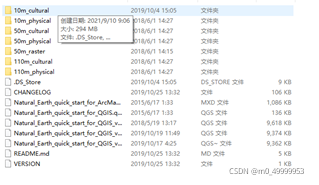# An error occurs when cartopy library uses the coastlines() command

Reinstall the system, resulting in the need to reconfigure python. Python version 3.7.9 and cartopy version 0.19.0.post1 are used

Example code:

``````import numpy as np
import cartopy.crs as ccrs
import matplotlib.pyplot as plt
import matplotlib.ticker as mticker
from cartopy.mpl.ticker import LongitudeFormatter,LatitudeFormatter
dlon,dlat=60,30
xticks=np.arange(0,360.1,dlon)
yticks=np.arange(-90,90.1,dlat)
fig=plt.figure(figsize=(6,5))
ax.coastlines()
gl=ax.gridlines(crs=ccrs.PlateCarree(),draw_labels=False,linewidth=1,linestyle=":",color="k",alpha=0.8)
gl.xlocator=mticker.FixedLocator(xticks)
gl.ylocator=mticker.FixedLocator(yticks)
ax.set_xticks(xticks,crs=ccrs.PlateCarree())
ax.set_yticks(yticks,crs=ccrs.PlateCarree())
ax.xaxis.set_major_formatter(LongitudeFormatter(zero_direction_label=True))
ax.yaxis.set_major_formatter(LatitudeFormatter())
plt.show()``````

The first time you use this command, you need to download the corresponding coalistline file, but there is a problem with the website in the program and you can’t download it. You need to download the file yourself on the earthnatural official website.Place the file in C: \ users \ XXXX \. Local \ share \ cartopy \ shapefiles \ natural_ Under the earth \ physical path, if the program still reports an error when running, you need to delete the path (. Local \ share \ cartopy \ shapefiles \ natural_earth \ physical) and run it again. After generating the path of the same path, copy the downloaded physical into physical, run it again, and no error will be reported. Code reference Python meteorological drawing tutorial (VII) — cartopy